Continuous Piecewise Linear Functions

We have already study a basic example of piecewise defined functions: step functions.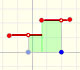A piecewise function is a function that is defined by several subfunctions. If each piece is a constant function then the piecewise function is called Piecewise constant function or Step function.

Now we are going to study piecewise functions where the pieces are not necessarily constant but linear. To start with we are going to consider only continuous piecewise linear functions. There is no discontinuity at each endpoint of the subdomains within that interval.

Continuous piecewise linear functions F and step functions f form pairs in some way.

A typical use of continuous piecewise linear functions is when we link several points in a graph using segments. This kind of approximation to a curve is known as Linear Interpolation.

Example of a continuous piecewise linear function is the definition of the absolute value function.We can consider now the derivative of each piece (in this case, the derivative is the slope of the line) and we get a an step function.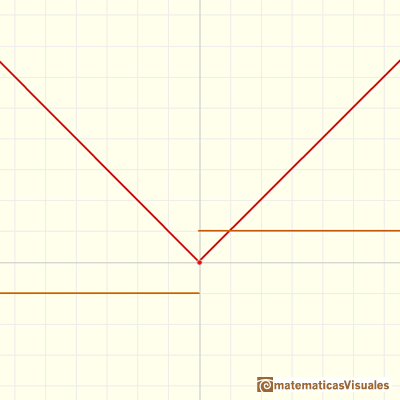Continuous piecewise linear functions are 'smooth almost everywhere'. There are only some points where the function is not smooth. They are examples of piecewise differentiable functions.

A function is piecewise differentiable if each piece is differentiable throughout its subdomain, even though the whole function may not be differentiable at the points between the pieces.

We remember that the graph of a linear function is an straight line.

The derivative of a linear function is a basic example of derivative, it is a constant function.The derivative of a lineal function is a constant function.

The integral of a non-constant linear function is a quadratic function.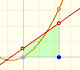It is easy to calculate the area under a straight line. This is the first example of integration that allows us to understand the idea and to introduce several basic concepts: integral as area, limits of integration, positive and negative areas.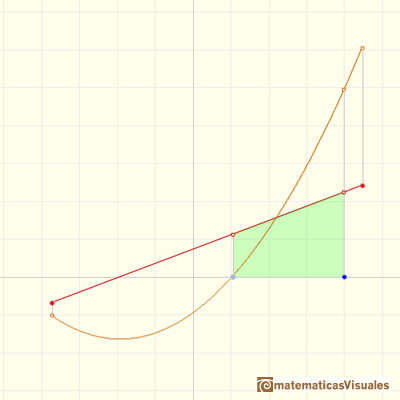Now we are going to study the relation between a continuous piecewise linear function F and the step function f build with the slopes.

We can play with the applet and start with a function F: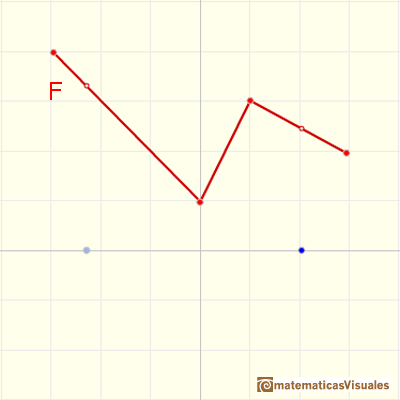Then the slopes define an step function f (you can see this function as velocity):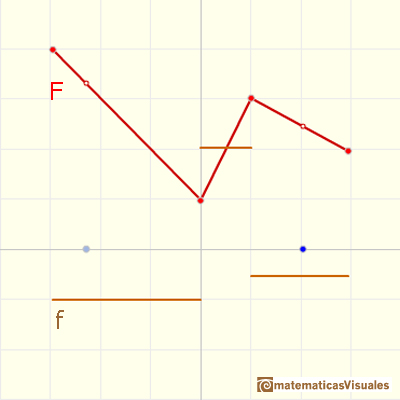If you consider the area under this step function f ...... you get a continuous linear piecewise function that is a vertical translation of the original piecewise function.This process is called integration. The function you get is one integral function (you can see it as distance, if f is velocity).

Changing the origin of integration (blue point, to the right and left) you can translate the integral function up and down.

In the next applet, we start with an step function f and look for one continuous linear piecewise function F that have slopes equal to the initial step function. As you know, this process is integration.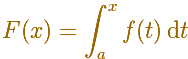You can drag up and down the blue dot to translate the integral function: there are infinite integral functions.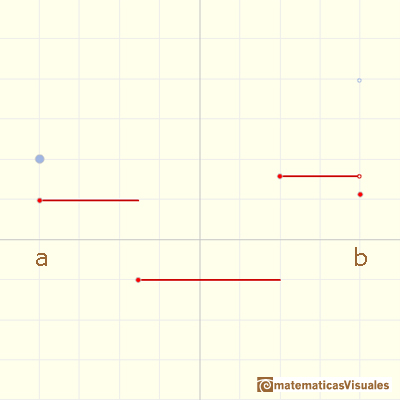If F(a) is fixed you can consider the function F(x)Suppose that you are asked about the value of F(b) and that the slopes of the different pieces are given.

Then you can calculate the values F(c), F(d) and F(b) using the point-slope formula for lines.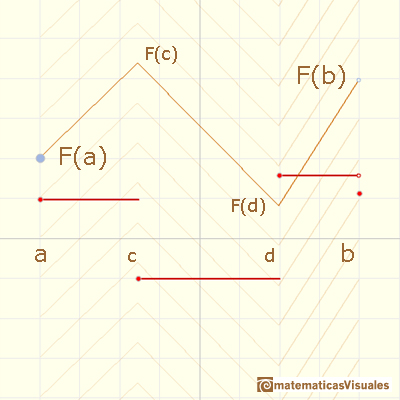If there is a lot of pieces, you have to work hard to get F(b).

F(x) is an integral function. Then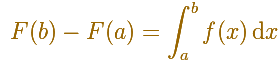This is the Fundamental Theorem of Calculus.

You can calculate the area piece by piece to get the integralOr you can use the average value and remember thatNow, this formula relates the Fundamental Theorem of Calculus with the point-slope formula:We are going to integrate a continuous piecewise linear function.The integral function F(x) is made by several connected pieces of parabola.You can see that the connection of these pieces of parabola is smooth.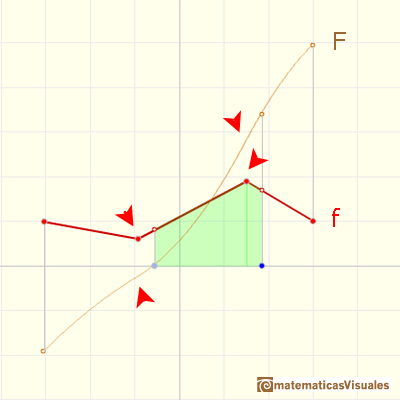This function F(x) is not only continuous, it is differentiable. You can see that dragging the blue dot and moving the tangent. It changes smoothly. This is a general property: when f continuous then F is more than continuous, it is differentiable.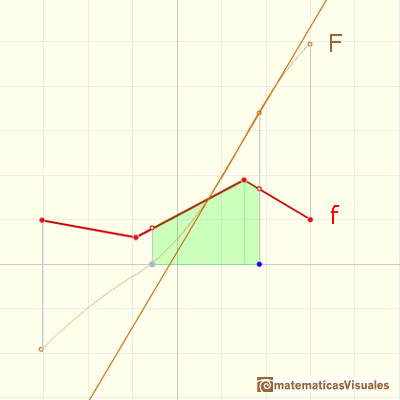If we differentiate F we get the original function f again.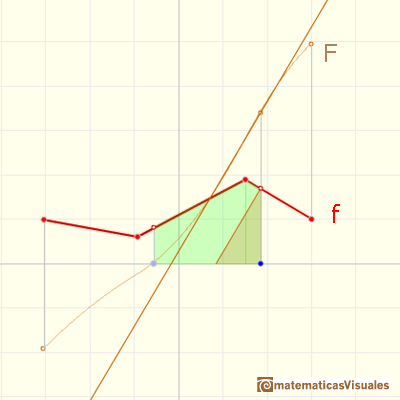The average value of a function f(x) over the interval [a,b] is given by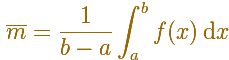In the next applet you can play with this concept.

As these functions are continuous, they are special cases of the Mean Value Theorem for integrals: If f(x) is a continuous function over the interval [a,b] then there is a number c in [a,b] such that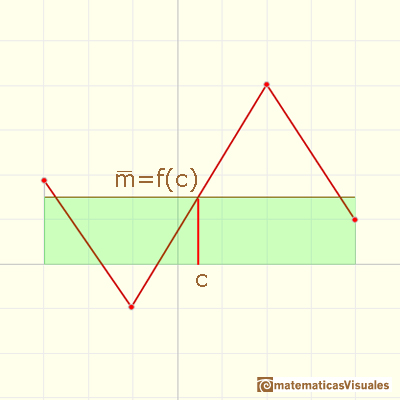Next we can study a more general case, discontinuous piecewise linear functions.

REFERENCES

Tom M. Apostol, Calculus, Second Edition, John Willey and Sons, Inc.
Gilbert Strang, Sums and Differences vs. Integrals and Derivatives, The College Mathematics Journal, January 1990. JSTOR.
Anthony J. Macula, The Point-Slope Formula Leads to the Fundamental Theorem of Calculus, The College Mathematics Journal, Mathematical Association of America, 1995.
Michael Spivak, Calculus, Third Edition, Publish-or-Perish, Inc.As an introduction to Piecewise Linear Functions we study linear functions restricted to an open interval: their graphs are like segments.Two points determine a stright line. As a function we call it a linear function. We can see the slope of a line and how we can get the equation of a line through two points. We study also the x-intercept and the y-intercept of a linear equation.Power with natural exponents are simple and important functions. Their inverse functions are power with rational exponents (a radical or a nth root)Polynomials of degree 2 are quadratic functions. Their graphs are parabolas. To find the x-intercepts we have to solve a quadratic equation. The vertex of a parabola is a maximum of minimum of the function.Polynomials of degree 3 are cubic functions. A real cubic function always crosses the x-axis at least once.We can consider the polynomial function that passes through a series of points of the plane. This is an interpolation problem that is solved here using the Lagrange interpolating polynomial.The derivative of a lineal function is a constant function.The derivative of a quadratic function is a linear function, it is to say, a straight line.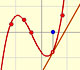The derivative of a cubic function is a quadratic function, a parabola.Lagrange polynomials are polynomials that pases through n given points. We use Lagrange polynomials to explore a general polynomial function and its derivative.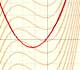If the derivative of F(x) is f(x), then we say that an indefinite integral of f(x) with respect to x is F(x). We also say that F is an antiderivative or a primitive function of f.It is easy to calculate the area under a straight line. This is the first example of integration that allows us to understand the idea and to introduce several basic concepts: integral as area, limits of integration, positive and negative areas.To calculate the area under a parabola is more difficult than to calculate the area under a linear function. We show how to approximate this area using rectangles and that the integral function of a polynomial of degree 2 is a polynomial of degree 3.The integral of power functions was know by Cavalieri from n=1 to n=9. Fermat was able to solve this problem using geometric progressions.We can see some basic concepts about integration applied to a general polynomial function. Integral functions of polynomial functions are polynomial functions with one degree more than the original function.The integral concept is associate to the concept of area. We began considering the area limited by the graph of a function and the x-axis between two vertical lines.Monotonic functions in a closed interval are integrable. In these cases we can bound the error we make when approximating the integral using rectangles.If we consider the lower limit of integration a as fixed and if we can calculate the integral for different values of the upper limit of integration b then we can define a new function: an indefinite integral of f.The Fundamental Theorem of Calculus tell us that every continuous function has an antiderivative and shows how to construct one using the integral.The Second Fundamental Theorem of Calculus is a powerful tool for evaluating definite integral (if we know an antiderivative of the function).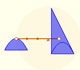Archimedes show us in 'The Method' how to use the lever law to discover the area of a parabolic segment.